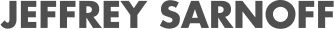# Fractional Order Computing and Modeling with Julia

07/29/2022, 12:30 PM1:00 PM UTC
Red

#### Abstract:

As the generalization of classical calculus and differential equations, fractional calculus and fractional differential equations are important areas since their invention, to provide a comprehensive Differential Equations package, SciFracX is here to explore fractional order area with Julia. In 2022 JuliaCon, we will talk about the progress we have made in FractionalDiffEq.jl and FractionalCalculus.jl, how Julia helped us speed up fractional order modeling and com

#### Description:

In 1695, a letter from L'Hopital to Leibniz represented the birth of the fractional calculus, "Can the meaning of derivatives with integer order be generalized to derivatives with non-integer orders? What if the order will be 1/2?", Leibniz replied in September 30, 1965: "It will lead to a paradox, from which one day useful consequences will be drawn".

Fractional order computing and modeling has become a more and more appealing topic especially recent decades, natural models usually can be more elaborated in fractional order area. Dating back to Leibniz and L'Hopital raised the "non-integer" calculus question, many giants of science have make hard work on fractional calculus to promote its further development. Fractional calculus are very helpful in describing linear viscoelasticity, acoustics, rheology, polymeric chemistry, and so forth. Moreover, fractional derivatives have been proved to be a very suitable tool for the description of memory and hereditary properties of various materials and processes.

SciML organization has done outstanding work in numerical solvers for differential equations, but there are still some kinds of differential equations that SciML has not supported yet, as the generalization of integer order differential equations, fractional calculus and fractional differential equations began to attract increasing interest for its important role in science and engineering since early 20th century. While most of the numerical software are programmed using Matlab and are not well maintained, users didn't have unifying tools to help with fractional order modeling and computing, being inspired by SciML, we initiated SciFracX organization. Our mission is to make the fractional order computing and modeling more easier using Julia, speed up researches and provides powerful scientific researching tools. Right now, there are four Julia packages in this organization: FractionalSystems.jl, FractionalCalculus.jl, FractionalDiffEq.jl and FractionalTransforms.jl. We would introduce the FractionalSystems.jl, FractionalDiffEq.jl and FractionalCalculus.jl packages.

The FractionalSystems.jl package is a package focus on fractional order control, inspired by FOMCON and FOTF toolbox, FractionalSystems.jl aims at providing fractional order modeling with Julia. Building on ControlSystems.jl, FractionalSystems.jl provides similar functionalities with ControlSystems.jl, mainly time domain and frequency domain modeling and analysis. While FractionalSystems.jl is only several months old, there are still a lot we need to do in the future.

The FractionalDiffEq.jl package follows the design pattern of DifferentialEquations.jl. To solve a problem, we first define a `***Problem` according to our model, then pass the defined problem to `solve` function and choose an algorithm to solve our problem.

``````prob = ***Problem(fun, α, u0, T)
#prob = ***Problem(parrays, oarrays, RHS, T)
solve(prob, h, Alg())
``````

Now, FractionalDiffEq.jl is capable of solving fractional order ODE, PDE, DDE, integral equations and nonlinear FDE systems, what impressed us the most is two times speedup compared to MATLAB when we were trying to solve the same problem in Julia(Didn't use any performance optimization)

FractionalCalculus.jl has supports for common sense of fractional derivative and integral, including Caputo, Riemann Liouville, Hadamard and Riesz sense etc. To keep it simple and stupid, we use two intuitive function `fracdiff` for fractional derivative and `fracint` for fractional integral. All we need to do is to pass the function, order, specific point, step size and which algorithm we want to use.

``````fracdiff(fun, α, point, h, Alg())
``````
``````fracint(fun, α, point, h, Alg())
``````

By using Julia in fractional order computing and modeling, we indeed observed amazing progress in no matter the speed or the ease of use in fractional order modeling and computing.

While there are no such similar organizations in Python or R community doing high performance computing in fractional order area, the future of SciFracX is promising, we envision a bright future for using Julia in fractional order area. Our work of next round is clear:

• Keep adding more high performance algorithms.
• Make the usage of API more simple and elegant.
• Write more illustrative documents for usability.
• Integrate with the SciML ecosystem to provide users more useful features.## Media partners## Community partners